Contents >> Engineering Mathematics >> Hydraulic Systems >> Dynamic Analysis >> Forming and solving algorithms of hydraulic circuit mathematical model

 Dynamic analysis of hydraulic systems - Forming and solving algorithms of hydraulic circuit math model Forming and solving algorithms of hydraulic circuit math model Forming of input data. All input information for carrying out of dynamic analysis of circuit of  hydraulic drive consists of two parts: - information on structure of the considered hydraulic circuit; - information about physical and design data of the hydraulic circuit elements. The first part of the input information is formed according to the method of the structure description of hydraulic circuits stated above, based on classification of base hydraulic elements, introduction of the generalized three-node element with nodes i (input), j (output) and k (control, transformation of energy, supply or taking away of power). On a basis of the entered classification each hydraulic element has received the certain identifier е , designating type of element and unequivocally defining group of equations for mathematical description of the given type element. According to it special forms (tables) of input data have been developed. Each element (except for directional control valves) is described by the line of table of input data having the following structure:(38) where n – number of element; А – matrix-line of coefficients describing physical, geometrical and design data of the element that makes the maintenance of the second part of the input information. At elements having only two nodes (for example, throttle, pipeline, diesel engine) the third node is designated by zero. Number of element n is intended to choice the necessary array of factors from general field of input data at forming of system of equations. As already it was marked above, the unique element which is dropping out of the concept of a three-node element is a directional control valve as a number of nodes adjoining it can be more than three. Therefore its description differs from other hydraulic elements. After numbering nodes of directional control valve it is necessary to write down in pairs numbers of nodes of its channels in various positions of spool, and the order of following of nodes (input-output) for each channel of spool is defined by direction of flow of working liquid. Thus change of direction of flow at change of position of spool can occur not in whole circuit. For example, in pressure head and drain lines of the directional control valve the direction of flow does not vary. Therefore for account of the direction of flow in node for each connection alongside with number of node it is necessary to specify a sign on flow in this node which is defined by the accepted positive direction of flow in a pipeline adjoining the given node. Each connection of nodes of directional control valve represents local resistance with variable area of through passage section depending on position of spool (Fig. 4). In view of these features the tables for directional control valves with other structure have been developed: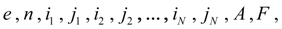(39) where е – the identifier of directional control valve; n – number of the directional control valve;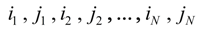– numbers of nodes of input ( i ) and output ( j ) of connections 1..., N ; A –  matrix-line of coefficients describing physical and design data of the directional control valve; F – a matrix of areas of through passage sections of connections 1..., N as function of the directional control valve spool movement z , and besides z is function of time, or function of some parameter (for example, pressure or movement of hydraulic cylinder piston, etc.). Representation of input data in such kind allows to make the uniform description of the circuit as switching of a spool from one position in another will automatically define the scheme of connections of elements. For forming of mathematical model of hydraulic system dynamics it is necessary to give also parameters of a working liquid entering into the equations ( density , kinematic viscosity , volumetric module of elasticity ). Forming of mathematical model of hydraulic system as a whole. As a result of analysis of line of the table of input data for each element depending on its identifier the system of differential and-or algebraic equations of a kind is formed: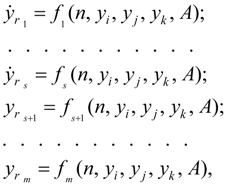(40) where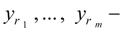generalized variables in nodes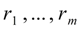; т – dimension of system of equations of the given type element; s – dimension of system of differential equations;accept values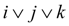, i.e. numbers of the nodes corresponding the given element; the concrete kind of right parts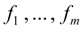, physical sense of variables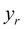, dimensions m and s are unequivocally defined by the identifier of element. For directional control valves the analysis of connections of its nodes is preceded with forming of the equations, as in intermediate position of a spool (at its switching from one position in another) in one node some connections can be crossed. Therefore flows in nodes belonging simultaneously various connections, turn out summation of flows in corresponding connections [see the equations (22), being analogue of the Kirchhoff’s first law for electric circuits]. The description of each hydraulic element is realized generally by two separate blocks: D (differential equations of mathematical model of element) and-or A (algebraic or transcendental equations of mathematical model of element). On a basis of input information the following fields of input data are formed. Matrix of communications: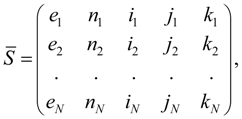(41) differing matrix S [see (1)] presence of an additional columnof numbers of elements. Matrix of parameters of elements of hydraulic system:(42) where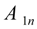– arrays of parameters of pumps;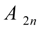– arrays of parameters of hydraulic motors;– arrays of parameters of hydraulic cylinders etc.;– arrays of parameters of directional control valves; М – number of types of elements in considered classification (can replenish); n =1, 2, … – numbers of the given type elements. Forming of mathematical model of hydraulic system is carried out on the following algorithm. The element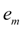( m = 1..., N ) is considered, its comparison with each of possible identifiers (PUMP, MOTOR, PIPE, ...) is made. At concurrenceto one of them the reference to the block of differential ( D ) and-or algebraic ( A ) equations is made with transfer of specification: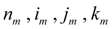. By meansfrom matrix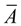its element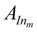(a line of factors) is selected, where I corresponds to the hydraulic element identifier defined before it. As a result the required equations in which the necessary coefficients are substituted, get out of the general library of mathematical models. Then there is a transition to a following element, etc. until the list of the hydraulic circuit elements (column е of matrix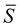) will be completely settled, that means the end of forming process of system of equations. External influences and control signals. Control of model (the system of the equations solving) is carried out by the special block which function includes generating signals of external influence: forces of resistance, external loadings in separate elements of hydraulic system (hydraulic motors, hydraulic cylinders), switching of operating hydraulic elements (spools of directional control valves, operated valves, adjustable throttles, pumps, etc.); stop of solving on the given condition, etc. In the control block it is possible to simulate as typical external influences (sine wave, intermittent, linear, etc.), and non-standard, taking place in most cases and defined by a specific problem. Moreover, for consideration of problems with changing physical and design data (variable masses and moments of inertia, pressures of adjustment of valves, etc.) in the control block it is possible to provide the task of these values as function of time or other variable received as a result of solution. Besides in the control block the forming of operating influences and forces of resistance as the functions approximated by a final set of discrete values or having a certain spectrum is obviously possible. Algorithm of solving. For integration of systems of the differential equations now there is a huge set of methods of numerical integration (Runge-Kutta, Euler’s, Gear's  methods and their updating, etc.), on a basis of which a majority of various corresponding programs has been developed that allows to choose the suitable tool of the solution always. However, in our case of simulation of dynamics of hydraulic systems there is a feature, consisting that the received system of the equations – the mixed type, alongside with differential it contains also algebraic (transcendental) equations for definition of flows of pumps, hydraulic motors, losses of pressure on length of pipelines, polytropic process in a gas cavity of the hydro pneumatic accumulator, various nonlinearity. The solution of such systems of the equations in the closed form (analytically) is not obviously possible because of insuperable complexities of transformations necessary for it. Therefore for search of the numerical solution it is possible to act as follows. Having values of pressure on input of the pipeline on n -th step of integration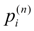, pressure upon outputwe’ll define, using the approached equation:(43) i.e. having taken values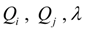on previous ( n – 1)-th step of integration. In view of small step of numerical integration, and also small all second addendum composed in the right part (43) an error received at such replacement, is negligible small. By virtue of the stated reasons the equation of losses of pressure on length of the pipelines, written down in the form of (43), yields quite satisfactory results and allows to solve other equations (for definition of flows) in a finite kind.

 < Previous Contents Next >